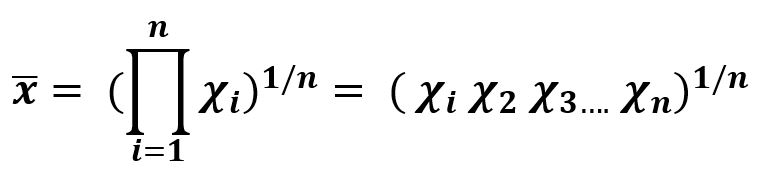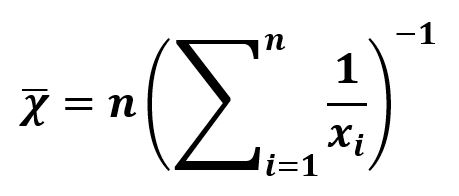# Understanding Types of Mean | Set 2

It is one of the most important concepts of statistics, a crucial subject to learn Machine Learning.

• Geometric Mean: Like arithmetic mean is the sum of all the discrete values in the set, Geometric mean is the product of discrete values in the set. It is useful for the set of positive discrete values.Example –

```Sequence = {1, 3, 9}

product         = 27
n, Total values = 3
Harmonic Mean   = (27)^(1/3)
```

Code –

 `# Geometric Mean  ` ` `  `import` `numpy as np ` ` `  `# discrete set of numbers ` `from` `scipy.stats.mstats ``import` `gmean ` `x ``=` `gmean([``1``, ``3``, ``9``]) ` ` `  `# Mean  ` `print``(``"Geometric Mean is :"``, x)  `

Output :

`Geometric Mean is : 3`
• Harmonic Mean : Harmonic mean plays it roles when it comes to calculate the mean of the terms which are in defined in relation to any unit. It is the reciprocal of the mean of the reciprocals of the data. Itis used where inverse variation in relation is involved in the data.Example –

```Sequence = {1, 3, 9}

sum of reciprocals = 1/1 + 1/3 + 1/9
n, Total values    = 3
Harmonic Mean      = 3 / (sum of reciprocals)
```

Code –

 `# Harmonic Mean  ` ` `  `import` `numpy as np ` ` `  `# discrete set of numbers ` `from` `scipy.stats.mstats ``import` `hmean ` `x ``=` `hmean([``1``, ``3``, ``9``]) ` ` `  `# Mean  ` `print``(``"Harmonic Mean is :"``, x)  `

Output :

`Harmonic Mean is : 2.076923076923077`
• Relationship between Arithmetic (AM), Harmonic (HM) and Geometric Mean (GM):Example –

```Sequence = {1, 3, 9}

sum of reciprocals = 1/1 + 1/3 + 1/9
Sum                = 10
Product            = 27
n, Total values    = 3

Arithmetic Mean = 4.33
Geometric Mean  = 3
Harmonic Mean   = 3 / (sum of reciprocals) = 2.077

```

Attention geek! Strengthen your foundations with the Python Programming Foundation Course and learn the basics.

To begin with, your interview preparations Enhance your Data Structures concepts with the Python DS Course.

My Personal Notes arrow_drop_upAspire to Inspire before I expire

If you like GeeksforGeeks and would like to contribute, you can also write an article using contribute.geeksforgeeks.org or mail your article to contribute@geeksforgeeks.org. See your article appearing on the GeeksforGeeks main page and help other Geeks.

Please Improve this article if you find anything incorrect by clicking on the "Improve Article" button below.